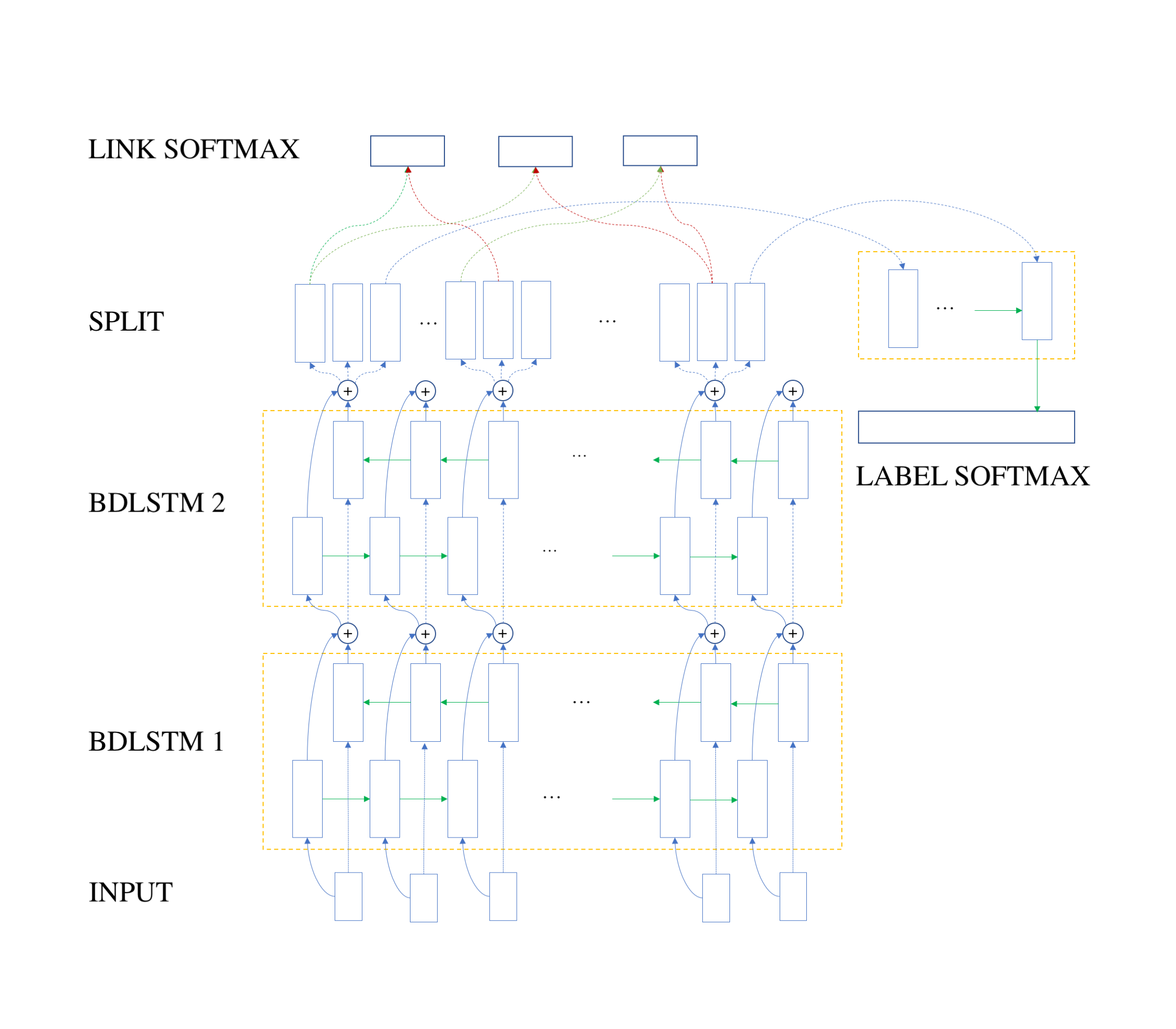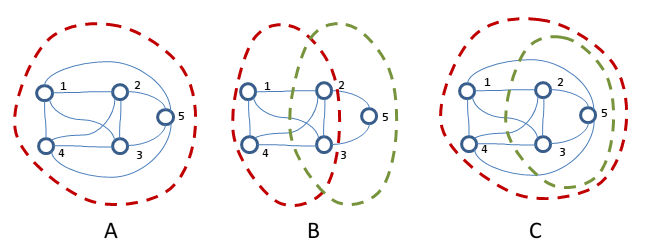# NLP - Cube

Sentence Splitting, Tokenization, Lemmatization, Part-of-speech Tagging, Dependency Parsing and Named Entity Recognition for more than 50 languages

# Graph-based decoding combined with deeplearning for named entity recognition

Multi-word expression (MWE) detection is a challenging Natural Language Processing (NLP) task that consists in finding isolated tokens or sequences of tokens that form high-level structures (i.e. multi-word expressions). Naively, this can be regarded as a sequence labeling task, which in fact influenced legacy MWE methods to use this strategy. In fact, this is not far fetched, since this approach has yielded highly accurate results so far. However, we have to point a couple of task-specific details:

• Sparsity: Inside an utterance there are only a couple of tokens that must be labeled as named entities or multi-word expressions. This actually yields data sparsity and could bias the model towards not identifying any of the tokens as part of a multi-word expression;
• Overlapping: MWE corpora contains many examples where high-level entities share tokens among each other. There are several ways in which this issue can be mitigated, for instance training several different classifiers for each type of label;
• Long spans: It is often the case that MWE tokens are distantly distributed across a single utterance. Classical classifiers and even modern LSTM-based approaches are unable to detect such long range dependencies (though the later mentioned approach should), mainly because these cases are rare inside the training data and there is insufficient statistical evidence to support them.

Our Named Entity Recognition (NER) system employs Graph-Based-Decoding (GBD) over a hybrid network architecture composed of bidirectional LSTMs for word-level encoding, with a MultiLayerPerceptron for detecting links inside entities and a unidirectional LSTM for label estimation:We are currently working on integrating the NER branch into master. Until then, you can check out the source for the GDB-NER system at this repository, see the results obtained on the PARSEME corpus here. If interested, we also encourage you to read our paper draft

Our graph-based encoding/decoding strategy is able to cope with overlapping high-level entities and large spans between tokens, by forcing the model to focus on the dependencies between tokens that belong to the same expression. We start off with a sequence of words as input, and convert it into a sequence of fixed-sized vectors (by combining lexical and morphological attributes for every word). After this, we use two stacked Bidirectional LSTM (BDLSTM) layers, obtaining a list of vector embeddings for each word inside the original sequence. Next, we take each vector from the internal representation and we split it using three parallel $tanh$ layers into 3 separate "projections".

As such, for each word $$w_i$$, we obtain three vectors which we will refer to as $$v_i^0$$, $$v_i^1$$ and $$v_i^2$$. Finally, for every pair of words $$w_i$$ and $$w_j$$ with $$i\neq j$$ we use single unit sigmoid activation, outputting the probability of the two words being part of the same high-level expression or not. The input for the sigmoid unit is obtained by concatenating the first vector from the split operation for word $$w_i$$ (i.e. $$v_i^0$$) with the second vector of the split operation for word $$w_j$$ (i.e. $$v_j^1$$). We store the resulting values in an adjacency matrix $$a$$.

Finally, we use a graph-based algorithm to decode NERs and assign the label using an unidirectional LSTM over the third projection ($$v_i^2$$) of the words contained in each expression (in natural order). Here we have to handle three cases:• The simplest Case (A) shows 5 interconnected graph nodes, which form a single high-level entity. Most of training data contains only this type of example, where we have to mark all the discovered tokens with the same label.
• Case (B) is more complex, as we have two overlapping high-level entities which share nodes 2 and 3. As such, the decoding algorithm can still easily spot the two distinct complete subgraphs, [1, 2, 3, 4] and [2, 3, 5] and mark them as two distinct entities (even if they are, or not, of the same type).
• Case (C) is by far the hardest. Here we have two distinct entities, with one entity [1, 2, 3, 4, 5] completely including the second entity [2, 3, 5]. We have not encountered this case in any of the training data but we feel obliged to discuss it as well. Obviously, given that the input data is correct, the decoding algorithm will always find a single entity, because all the nodes are adjacent and there is no reason to perform a split into two subgraphs. One solution to this issue is to check if any partial subgraph yields a different label then the full subgraph. This solution is only valid for entities which belong to different classes, but we feel that the two expressions sharing the same label would be highly unlikely to overlap. However, this solution may not always hold, and in the absence of real data to support Case (C).